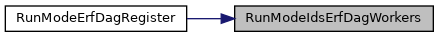suricata
runmode-erf-dag.h File Reference
This graph shows which files directly or indirectly include this file: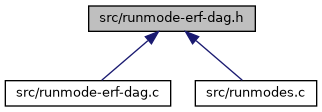Go to the source code of this file.

Functions

int RunModeIdsErfDagAutoFp (void)

int RunModeIdsErfDagSingle (void)

int RunModeIdsErfDagWorkers (void)

void RunModeErfDagRegister (void)

const char * RunModeErfDagGetDefaultMode (void)

Function Documentation

 const char* RunModeErfDagGetDefaultMode ( void )

Definition at line 45 of file runmode-erf-dag.c.

Referenced by RunModeDispatch().

Here is the caller graph for this function:void RunModeErfDagRegister ( void )

Definition at line 50 of file runmode-erf-dag.c.

Referenced by RunModeRegisterRunModes().

Here is the call graph for this function: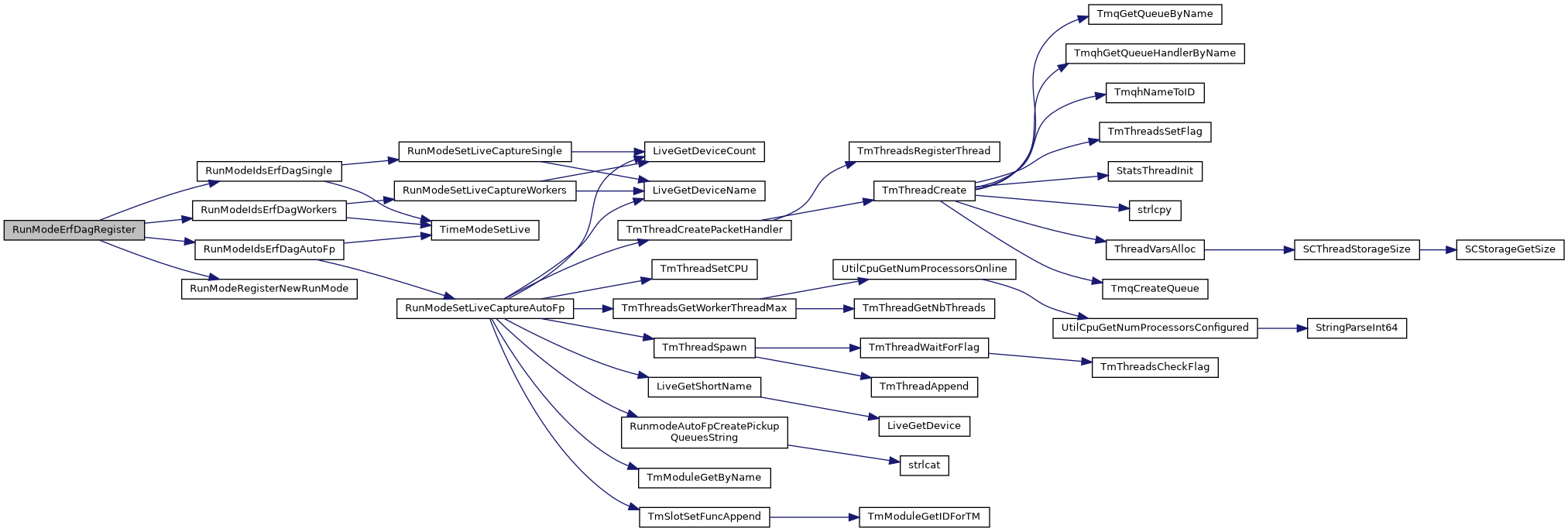Here is the caller graph for this function:int RunModeIdsErfDagAutoFp ( void )

Definition at line 100 of file runmode-erf-dag.c.

Referenced by RunModeErfDagRegister().

Here is the call graph for this function: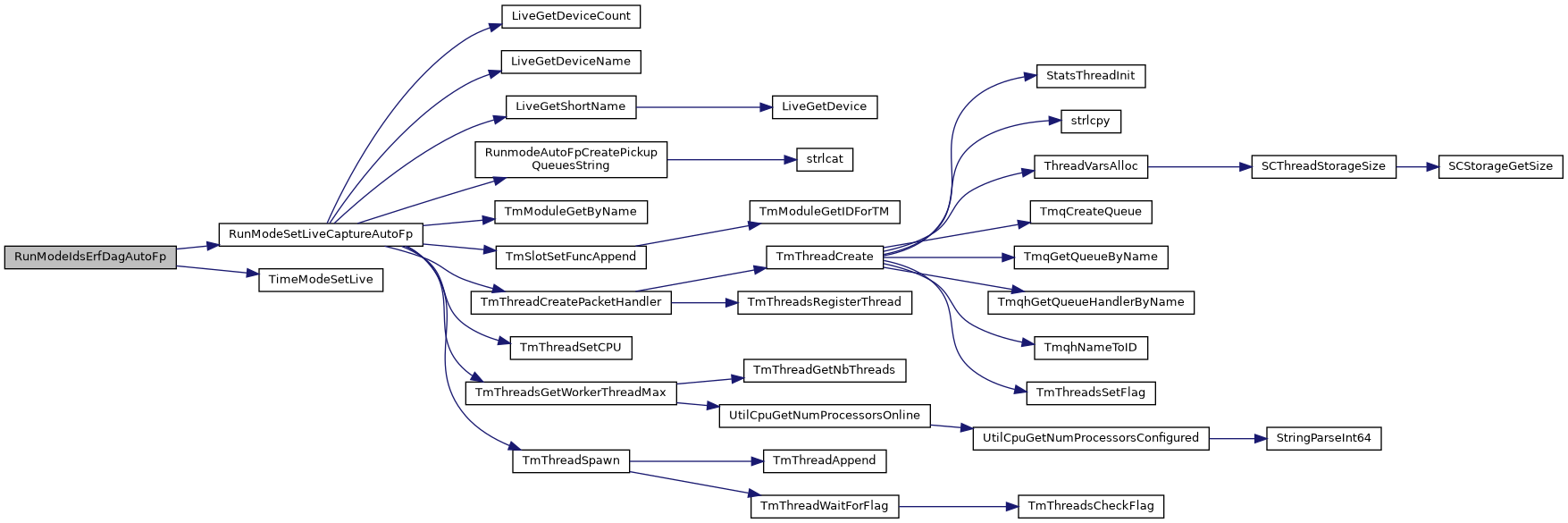Here is the caller graph for this function: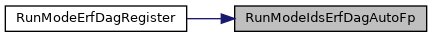int RunModeIdsErfDagSingle ( void )

Definition at line 74 of file runmode-erf-dag.c.

Referenced by RunModeErfDagRegister().

Here is the call graph for this function: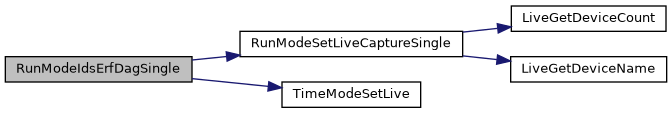Here is the caller graph for this function: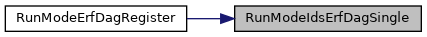int RunModeIdsErfDagWorkers ( void )

Definition at line 126 of file runmode-erf-dag.c.

Referenced by RunModeErfDagRegister().

Here is the call graph for this function: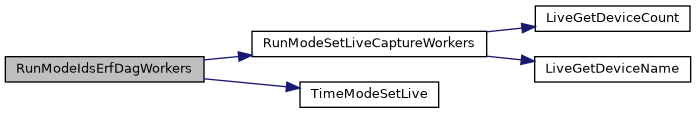Here is the caller graph for this function: